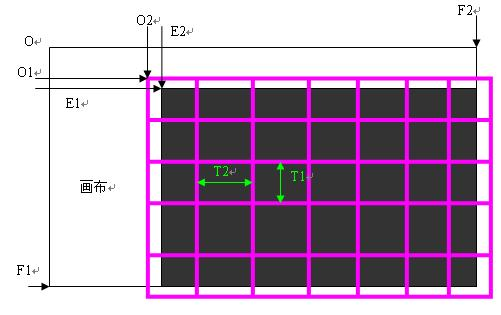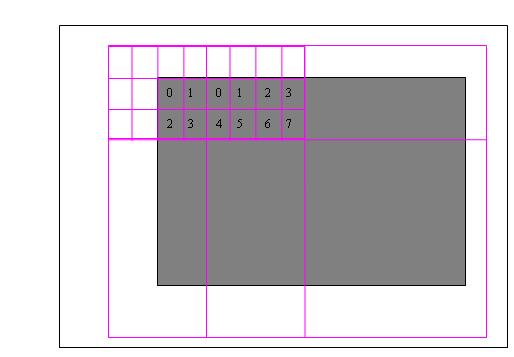# 学海苦作舟，书山勤为径

• 随笔 - 151
• 文章 - 0
• 评论 - 501
• 引用 - 0

• 积分 - 453112
• 排名 - 42

## JPEG2000中图像单元的定义

JPEG2000 中包括许多既定义的单元，主要有：

1．  画布

2．  分片（ Tile

3．  子带（ Subband

4．  分辨率层（ Resolution

5．  分区 (Precinct)

6．  分区分割（ Precinct partition, kakadu 中叫 precinct band

7．  码块（ code block

8．  (packet

9．  层（ layer

10．              分量（ Component

11．              区域（ Region

12．              渐进性（ Progressive

Ec = Ei/Si

# 贴片（分片）

JPEG2000 引入分片这个概念是为了处理复合文档，因为有些区域包含图形，有些区域包含文字，分片之后对不同的区域采用不同的处理方法。例如：文字区域不需要 DWT ，直接压缩等。

t = t2+t1N2

## 贴片在画布中的区域

E1_t = max (E1, O1+t1*T1); F1_t = min (F1, O1+t1*T1)

E2_t = max (E2, O2+t2*T2) F2_t = min (F2, O2+t2*T2)

O = 0 0

O1 = 10 O2 = 10 T1 = 100 T2 = 100

N1 = F1-O1 /100 = 9 N2  = 9

E1_t = max (100, 10+2*100) = 210 F1_t = min (1000, 10+3*100) = 310

E2_t = max (100, 10+2*100) = 210 F2_t = min (1000, 10+3*100) = 310JPEG2000 中， DWT 变换是针对每个贴片分量的，如果有三个分量，那么每个分量调用 DWT 变换。

# 子带

HL 子带是水平高通滤波器产生的结果，因此水平方向上高频图像区域被保存了下来。

LH 子带是垂直高通滤波器产生的结果，因此垂直方向上高频图像区域被保存下来。

HH 是垂直和水平方向上都是高通的结果，因此是对角线方向的图像特性被保存下来。

LL 是图像的低频部分，也是图像的低分辨率版本。

 LL3 HL3 HL2 HL1 LH3 HH3 LH2 HH2 LH1 HH1

## 子带在画布中的区域

E1_t_b = (E1_t – 2 d-1 b1)/2 d   E2_t_b = (E2_t – 2 d-1 b2)/2 d

F1_t_b = (F1_t- 2 d-1 b1)/2 d   F2_t_b = (F2_t- 2 d-1 b2)/2 d

LL 子带中： b1= b2= 0

HL 子带中： b1= 0 b2= 1

LH 子带中： b1= 1 b2= 0

HH 子带中： b1= 1 b2= 1

E1_t_b_LL = (210-2 2-1 *0)/2 2 = 210/4 = 53

E2_t_b_LL = 53

F1_t_b_LL = (310-2 2-1 *0)/2 2 = 78

F2_t_b_LL = 78

E1_t_b_HL= (210-2 2-1 *0/2 2 = 210/4 = 53

E2_t_b_HL= (210-2 2-1 *1)2 2 = 208/4 = 52

F1_t_b_HL = (310-2 2-1 *0)/2 2 = 78

F2_t_b_HL = (310-2 2-1 *1)/2 2 = 77

E1_t_b_LH= (210-2 2-1 *1/2 2 = 210/4 = 52

E2_t_b_ LH = (210-2 2-1 *0)2 2 = 208/4 = 53

F1_t_b_ LH = (310-2 2-1 *1)/2 2 = 77

F2_t_b_HL = (310-2 2-1 *0)/2 2 = 78

E1_t_b_ HH = (210-2 2-1 *1/2 2 = 210/4 = 52   E2_t_b_HH = 52

F1_t_b_ HH = (310-2 2-1 *1)/2 2 = 77              F2_t_b_ HH = (310-2 2-1 *1)/2 2 = 77

E1_t_b_LL = (210-2 1-1 *0)/2 1 = 210/2 = 105

E2_t_b_LL = 105

F1_t_b_LL = (310-2 1-1 *0)/2 1 = 155

F2_t_b_LL = 155

W_HL= F2_t_b_HL - E2_t_b_HL = 77-52 = 25

H_HL= F1_t_b_HL – E1_t_b_HL = 78-53 = 25

W_LH = F2_t_b_LH - E2_t_b_LH = 78-53 = 25

H_ LH = F1_t_b_ LH – E1_t_b_ LH = 77-52 = 25

W_HH = F2_t_b_HH –E2_t_b_HH = 77-52 = 25

H_HH = F1_t_b_HH – E1_t_b_HH = 77-52 = 25

W_LL = 78-53 = 25      H_LL = 78-53 = 25

E1_t_b_LL = (210-2 3-1 *0)/2 3 = 210/8 = 27

E2_t_b_LL = 27

F1_t_b_LL = (310-2 3-1 *0)/2 3 = 39

F2_t_b_LL = 39

E1_t_b_HL= (210-2 3-1 *0/2 3 = 210/8 = 27

E2_t_b_HL= (210-2 3-1 *1)2 3 = 206/8 = 26

F1_t_b_HL = (310-2 3-1 *0)/2 3 = 39

F2_t_b_HL = (310-2 3-1 *1)/2 3 = 39

E1_t_b_LH= (210-2 3-1 *1/2 3 = 206/8 = 26

E2_t_b_ LH = (210-2 3-1 *0)2 3 = 210/4 = 27

F1_t_b_ LH = (310-2 3-1 *1)/2 3 = 39

F2_t_b_ LH = (310-2 3-1 *0)/2 3 = 39

E1_t_b_ HH = (210-2 3-1 *1/2 3= 210/4 = 26   E2_t_b_HH = 26

F1_t_b_ HH = (310-2 3-1 *1)/2 3 = 39              F2_t_b_ HH = (310-2 2-1 *1)/2 2 = 39

LL 子带是： 12*12 HL 子带是 12*13 LH 13*12 HH 13*13 （高度先）。

LL 子带的位置为：（ 212 312 X 212 312

HL 子带的位置为：（ 212 312 X 208 308

LH 子带的位置为：（ 208 308 X 212 312

HH 子带的位置为：（ 208 208 X 208 308

# 分辨率层

## 分辨率的区域边界

E i _R = E i _t/2 D-r

F i _R = F i _t/2 D-r

E1_R_2 = 210/2 3-2 = 105      F1_R_2 = 310/2 3-2 = 155

E2_R_2 = 105      F2_R_2 = 155

E1_R_2 = 210/2 3-0 = 27        F1_R_2 = 310/2 3-0 = 39

E2_R_2 = 27        F2_R_2 = 39

# 分区

N1_P ：表示垂直方向分区的数量。

N2_P ：表示水平方向分区的数量。

0<=p1<N1_P   0<= p2<N2_P

N1_P N2_P 有下面计算得到：

N1_P = (F1_R-O1_P)/P1

N2_P = (F2_R-O1_P)/P2

F1_R F2_R 表示当前分辨率层的右和下边界。如果他们是 0 ，那么 N1_P N2_P 必须是 0

## 分区的区域

E1_P = max (E1_R, O1_P+P1*p1)

F1_P = min (F1_R, O1_P+P1*(p1+1))

E2_P = max (E2_R, O2_P+P2*p2)

F2_P = min (F2_R, O2_P+P2*(p2+1))

E1_P = max(105 90+1*8)= 105

F1_P = min (155,100+1*8) = 108

E2_P =max (105, 90+3*4) = 105

F2_P = min (155,100+3*4) = 112

# 分区分割

Ei_R-1_b = (Ei_R – bi)/2 Sr

Fi_R-1_b = (Fi_R – bi)/2 Sr

Sr r=0 的时候为 0 r 大于 0 的时候为 1 。如果是 0 表示最低分辨率层也就是最终的 LL 子带，那么其没有映射，而对于其他的分辨率层上的映射是其下一级别的三个子带，因此区域标记需要减少 1/2 。这里的关系同前面子带的映射关系相似，这里不在详细介绍。

Oi_R-1 = (Oi_R –bi)/2 Sr

P1_R-1 = 2 -Sr *P1_R

P2_R-1 = 2 -S r*P2_R

# 码块

J1_BC = MIN (J1_BC_D, 2 -Sr *P1_R)

J2_BC = MIN (J2_BC_D, 2 -Sr *P2_R)

P1_R P2_R 表示 r 分辨率上的分区大小。如果 2 -Sr *Pi_R Ji_BC_D 要小，说明一个分区只能有一个码块。

N1_BC = (F1_R-1_b – O1_P)/J1_BC

N2_BC = (F2_R-1_b – O2_P)/J2_BC

F1_R-1_b F2_R-1_b 表示分区在子带上的下边界和右边界。# 包JPEG2000 中有一个要求就是，将每个码块的头放在一起，组成包头，然后是码块的系数编码流。

# 渐进性

JPEG2000 最诱人的一点在于渐进性，随着数据的增加图像按照某种方式改善。

posted on 2006-12-27 21:55 笨笨 阅读(2117) 评论(2)  编辑 收藏 引用 所属分类: 压缩算法### 评论

#### #re: JPEG2000中图像单元的定义 2007-05-12 10:40 李

 只有注册用户登录后才能发表评论。 【推荐】超50万行VC++源码: 大型组态工控、电力仿真CAD与GIS源码库 相关文章: